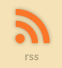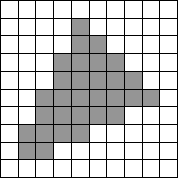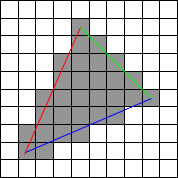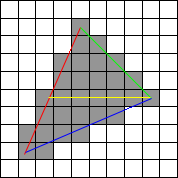Sol::Tutorials

# Triangle Rasterization for Dummies

Let's say you need to fill a triangle for some obscure reason. Hey, we have hardware for this kind of thing nowadays!Here you have a simple triangle that's been rasterized. This is what we'll want.

As input data we have three points like so. We'll call them A, B and C:When these three points get to you, they are most likely not in a nice order like above, so the first task is to sort them in order of their Y-coordinate. We'll get to the 'why' pretty soon.

``````
// find the lowest Y
if (vtx.y < vtx.y)
{
if (vtx.y < vtx.y)
{
order = 0;
}
else
{
order = 2;
}
}
else
{
if (vtx.y < vtx.y)
{
order = 1;
}
else
{
order = 2;
}
}

// find the highest Y
if (vtx.y > vtx.y)
{
if (vtx.y > vtx.y)
{
order = 0;
}
else
{
order = 2;
}
}
else
{
if (vtx.y > vtx.y)
{
order = 1;
}
else
{
order = 2;
}
}

// and the middle one is a matter of deduction
order = 3 - (order + order);
``````

Next thing to consider are the edges.Now, an easy way to do triangle drawing would be to simply make two arrays with the left and right coordinate and first "draw" these edges to those buffers, and then simply travelse the buffer. Here we'll do something slightly smarter and follow the left and the right edge at the same time.

Since the vertices are in order, we know how to produce the slopes for the two edges that we start with: from A to B and from A to C.

``````
d0 = (vtx[order].x - vtx[order].x) / (vtx[order].y - vtx[order].y);
d1 = (vtx[order].x - vtx[order].x) / (vtx[order].y - vtx[order].y);
``````

And the starting Y and starting left and right edges are naturally from vertex A. Now it's relatively simple to just loop scanlines until we hit vertex B, at which point we've drawn first half of our triangle.Next we'll just replace the correct slope (the A-B one) with the correct one (B to C) and do another loop until we hit vertex C.

``````
d0 = (vtx[order].x - vtx[order].x) / (vtx[order].y - vtx[order].y);
``````

Simple. Except that there are a couple special cases. As it happens, all three special casses are related to divisions by zero on the edge calculation.

If (A.Y - C.Y) is zero, the first division of zero would occur. In this case the polygon has no height at all, and you can return before you even get started.

If (B.Y - C.Y) is zero, we have a bottom-flat triangle, which is basically just the top half of the example triangle. In this case you're done when you hit B.Y.

Finally, if (A.Y - B.Y) is zero, we have a flat-top triangle, which is basically the bottom half of our example triangle. In this case we can skip rendering of the top triangle completely, but do remember to get the starting left and right edge values from the vertices A and B.

There's lots more to triangle rasterization, but these are the basics. Interpolate color between vertices for gouraud. Interpolate UV coordinates between vertices for (non-perspective) texturing. Play around with homogenious coordinates for more intesting stuff. Oh, and clipping is also a topic to itself.

Have fun!